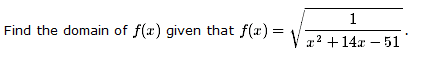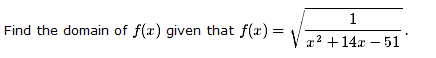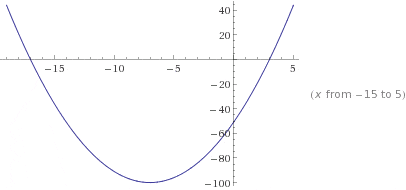# Finding the domain of a function (Sqr root)

• nukeman
In summary, the conversation discusses finding the domain of a function and solving a quadratic inequality. The domain is determined by considering the restrictions on the denominator and the values that result in a positive product when taking the square root. The final answer for the domain is (-17, 3) (3, +infinity).

## Homework Statement

Here is a picture of the question:## The Attempt at a Solution

Here it what I did...

factored it...so

1 / (x-3)(x+17)

domain: (-infinity, -3)(-3,17)(17, +infinity) or rather (-3,17)

I know that is not correct. I am really stuck on this one!

nukeman said:

## Homework Statement

Here is a picture of the question:## The Attempt at a Solution

Here it what I did...

factored it...so

1 / (x-3)(x+17)

domain: (-infinity, -3)(-3,17)(17, +infinity) or rather (-3,17)

I know that is not correct. I am really stuck on this one!

There are two things to consider here:
1) The denominator in the radical can't be zero, which happens when x = - 3 or x = 17
2) The denominator in the radical can't be negative. You have three intervals. Pick a number in each interval, to see if you get a negative value for the denominator. If you do, that interval is not in the domain.

Last edited:
So, is the domain just 17?

nukeman said:
So, is the domain just 17?
No, not at all. 17 is NOT in the domain, because f(17) is undefined. The domain is an interval or maybe two, of real numbers.

Evaluate x2 + 14x - 51 at any number from each of these intervals:

(-∞, -3)
(-3, 17)
(17, ∞)

If you get a negative result, that interval is NOT in the domain.
If you get a positive result, that interval IS in the domain.

Last edited:
Mark44 said:
No, not at all. 17 is NOT in the domain, because f(17) is undefined. The domain is an interval or maybe two, of real numbers.

Evaluate x2 + 14x - 51 at any number from each of these intervals:

(-∞, -3)
(-3, 17)
(17, ∞)

If you get a negative result, that interval is NOT in the domain.
If you get a positive result, that interval IS in the domain.

Oh right...

in the (-3,17) interval there are some numbers that give me a pos number.
Also in the interval (17, infinity)

so is it those 2 intervals? I am slightly confused

Last edited by a moderator:
nukeman said:

## Homework StatementOK !

Let's go back to the original problem.

As Mark44 said, there are two things to consider.

Mark44 said:
There are two things to consider here:
1) The denominator in the radical can't be zero, ...
2) The [STRIKE]denominator[/STRIKE] (quantity) in the radical can't be negative...
So,

(1): $x^2 + 14x - 51\ne 0$

and

(2): $x^2 + 14x - 51\ge 0$

Of course, that leaves you with $x^2 + 14x - 51>0\ .$

Can you solve that ?

Last edited by a moderator:
SammyS said:
OK !

Let's go back to the original problem.

As Mark44 said, there are two things to consider.

So,

(1): $x^2 + 14x - 51\ne 0$

and

(2): $x^2 + 14x - 51\ge 0$

Of course, that leaves you with $x^2 + 14x - 51>0\ .$

Can you solve that ?

To solve that would I try and isolate the x ?

Last edited by a moderator:
nukeman said:
To solve that would I try and isolate the x ?
It's an inequality, not an equation.

There are several ways to approach solving a quadratic inequality. I have no idea regarding which method(s) you may have previously learned.

SammyS, I also have had trouble solving inequalities. I am just getting back into the grove of things, and this was something I never really mastered unfortunately.

What is a good method? One that you use, or rather one you recommend a novice like my self?

From what I remember, I would just make it = to 0 then factor it.

Then I would draw a number line, and since it much be greater than 0, I would pick the one that is greater than 0

?

Last edited by a moderator:
nukeman said:
From what I remember, I would just make it = to 0 then factor it.

Then I would draw a number line, and since it much be greater than 0, I would pick the one that is greater than 0

?

wait...why did you change it from 51 to 17?
I suppose I looked at a post with a wrong number.

Yes, it should be $x^2 + 14x - 51>0\ .$

SammyS said:
I suppose I looked at a post with a wrong number.

Yes, it should be $x^2 + 14x - 51>0\ .$
That was my fault - I copied a number incorrectly. I have fixed it throughout this thread.

nukeman said:
Oh right...

in the (-3,17) interval there are some numbers that give me a pos number.
No, there aren't. Every number in this interval gives you a negative product from (x + 3)(x - 17).

Think about it: if -3 < x < 17, then x + 3 will be > 0, but x - 17 will be < 0. This gives you a negative product.
nukeman said:
Also in the interval (17, infinity)

so is it those 2 intervals? I am slightly confused

For the interval (17, ∞), all numbers produce a positive product.

What's the other interval that produces a positive product?

nukeman, you said, originally, that the function could be written
$$\sqrt{\frac{1}{(x- 3)(x+ 17)}}$$
and everything you need to know is in that. In order to take the square root the fraction inside the square root must be positive (it is never 0) and, since the numerator is always positive (it is always "1"), the denominator must be positive. But a product of numbers, like (x- 3)(x+ 17), is positive if and only if the two factors have the same sign. That is, they must both be positive or both negative.

So there are two possibilities:
1) x- 3> 0 and x+ 17> 0
or
2) x- 2< 0 and x+ 17< 0.

What values of x satisfy one of those two? (crucial point: -17< 3)

Last edited by a moderator:
It would be this one wouldn't it? x- 3> 0 and x+ 17> 0

Man, I got really confused on this one. SO SORRY, but can we start right at the start.

WHEN I see a question like this, let's take this exact one, what is the first thing I do?
I would factor it correct?

Last edited:
g(x)=x2+14x-51
Sketch the graph. It is a parabola
Check for x-intercept where g(x)=0.
Check the values of x where g(x)>0 and g(x)<0

f(x)=√1/g(x)
Domain of f(x) only if g(x)>0.

Last edited:
I am still not 100% on the final answer ?

(-17, 3) (3, +infinity)

nukeman said:

(-17, 3) (3, +infinity)
Let's see.

Did you graph $y=x^2 + 14x - 51$ as has been suggested?

Test some values of x ...

x = 0 is an easy one in the interval (-17, 3). Plugging in x=0 gives, y = -51 .

It turns out that any other value you might pick for x from (-17, 3) will give you a negative result for y.

Have you graphed $y=x^2 + 14x - 51\ ?$

Pick a value for x from (3, +∞) ...

x = 10 is fairly easy.

If x = 10 , $y=(10)^2 + 14(10) - 51=100+140-51=189$

It turns out that any other value you might pick for x from (3, +∞) will give you a positive result for y.

Have you graphed $y=x^2 + 14x - 51\ ?$

I don't understand the steps then :(

I look at the question: $y=x^2 + 14x - 51$

So, I factor it! ----> Then I get from factoring: (x - 3) (x +17)

When I get here. What do I do next?

SammyS said:
Let's see.

Did you graph $y=x^2 + 14x - 51$ as has been suggested?

Test some values of x ...

x = 0 is an easy one in the interval (-17, 3). Plugging in x=0 gives, y = -51 .

It turns out that any other value you might pick for x from (-17, 3) will give you a negative result for y.

Have you graphed $y=x^2 + 14x - 51\ ?$

Pick a value for x from (3, +∞) ...

x = 10 is fairly easy.

If x = 10 , $y=(10)^2 + 14(10) - 51=100+140-51=189$

It turns out that any other value you might pick for x from (3, +∞) will give you a positive result for y.

Have you graphed $y=x^2 + 14x - 51\ ?$

Wait, is the domain just (3, +infinity) ?

EDIT: Wait no.. is it:

(-infinity, -17) and (3, +infinity)

?

Last edited:
nukeman said:
Wait, is the domain just (3, +infinity) ?

EDIT: Wait no.. is it:

(-infinity, -17) and (3, +infinity)

?

Yes, it's (-∞, -17) (3, +∞)

Do you understand why?#### Attachments

Without looking at the graph, which makes it obvious...(now lol)

its because -17 to +3 are all negative numbers.

But, without making or looking at the graph of this function, and given the 2 sets (-17,3) how does one know that from -17 to 3 is all negative numbers?

nukeman said:
Without looking at the graph, which makes it obvious...(now lol)

its because -17 to +3 [STRIKE]are[/STRIKE] all give negative numbers.

But, without making or looking at the graph of this function, and given the 2 sets (-17,3) how does one know that from -17 to 3 is all negative numbers?
We tried to get you to look at the graph...

There are many ways to arrive at the solution. Here's one that uses what you tried to do with the inequalities:

From the factor (x -3) you got the result x > 3 . What this really tells you is the values of x which make the factor, (x - 3), positive. Of course, x = 3 makes (x - 3) zero, and x < 3 makes (x - 3) negative.

Similarly, from the factor (x + 17) you got the result x > -17. What this tells you is the values of x which make the factor, (x + 17), positive. Of course, x = -17 makes (x + 17) zero, and x < -17 makes (x + 17) negative.

You want the product of (x -3) times (x + 17) to be positive, so both factors must have the same sign. What values of x make both factors positive? What values of x make both factors negative?